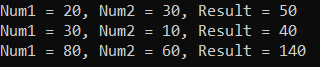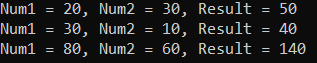# Static Local Functions in C#

## Static Local Functions in C# 8 with Examples

In this article, I am going to discuss Static Local Functions in C# 8 with Examples. Please read our previous article where we discussed Using Declarations in C# with Examples. Let us first understand what are local functions in C# and then we will understand the Static Local Functions in C#.

##### Local Function in C#

The Local Functions are introduced as part of C# 7. The local function allows us to declare a function inside the body of an already-defined function. In simple words, we can also say that a local function is a private function of a function whose scope is limited to the function where it is created. You can only call the local function from the parent function where it is created.

Let us see an example to understand the local functions in C#. In the below example, Calculate is the parent function and CalculateSum is the local function and this CalculateSum function can be called anywhere within the scope of Calculate function. From the main function, we can call the Calculate function, but we cannot call the CalculateSum function.

```using System;
namespace Csharp8Features
{
public class LocalFunctions
{
public static void Main()
{
Calculate();
//You cannot call the CalculateSum function
//CalculateSum();
}
public static void Calculate()
{
int X = 20, Y = 30, Result;
CalculateSum(X, Y);

// Here CalculateSum is the local function of the Calculate function
void CalculateSum(int Num1, int Num2)
{
Result = Num1 + Num2;
Console.WriteLine(\$"Num1 = {Num1}, Num2 = {Num2}, Result = {Result}");
}

// Calling Local function
CalculateSum(30, 10);
CalculateSum(80, 60);
}
}
}
```
###### Output:If you notice in the above example, the CalculateSum function is able to access the Result variable. This enables the usage of variables such as Result within the local method. If the usage is accidental, this might lead to huge consequences. To overcome this problem static local functions are introduced in C# 8.

##### Static Local Function in C# 8:

Local functions are introduced in C# 7. But in C# 7, it is not possible to use a static modifier with the local function i.e. static local functions are not allowed. This feature is added in C# 8.0. From C# 8.0, we are allowed to use a static modifier with the local function. This ensures that the static local function does not reference any variable from the enclosing or surrounding scope. If a static local function tries to access the variable from the enclosed scope, then the compiler will throw an error. Let us understand this with an example. Now, let us modify the same example by just adding the static modifier in the local function.

```using System;
namespace Csharp8Features
{
public class LocalFunctions
{
public static void Main()
{
Calculate();
//You cannot call the CalculateSum function
//CalculateSum();
}
public static void Calculate()
{
int X = 20, Y = 30, Result;
CalculateSum(X, Y);

// Here CalculateSum is the local function of the Calculate function
static void CalculateSum(int Num1, int Num2)
{
Result = Num1 + Num2;
Console.WriteLine(\$"Num1 = {Num1}, Num2 = {Num2}, Result = {Result}");
}

// Calling Local function
CalculateSum(30, 10);
CalculateSum(80, 60);
}
}
}
```

Now, you will get a compile-time Error CS8421 A static local function cannot contain a reference to ‘Result’.

With C# 8.0, .NET removed this limitation. This enables the developers to create a pure local function as it does not allow the usage of variables from enclosing types within it. For a better understanding, please have a look at the below code.

```using System;
namespace Csharp8Features
{
public class LocalFunctions
{
public static void Main()
{
Calculate();
//You cannot call the CalculateSum function
//CalculateSum();
}
public static void Calculate()
{
int X = 20, Y = 30;
CalculateSum(X, Y);

// Here CalculateSum is the local function of the Calculate function
static void CalculateSum(int Num1, int Num2)
{
int sum = Num1 + Num2;
Console.WriteLine(\$"Num1 = {Num1}, Num2 = {Num2}, Result = {sum}");
}

// Calling Static Local function
CalculateSum(30, 10);
CalculateSum(80, 60);
}
}
}
```

Now, if you notice we are not using any scope variables within the static local function CalculateSum. Now, run the above code and you will get the output as expected as shown in the below image.So, a static local function cannot capture the state from the enclosing scope. As a result, locals, parameters, and this from the enclosing scope are not available within a static local function in C#.